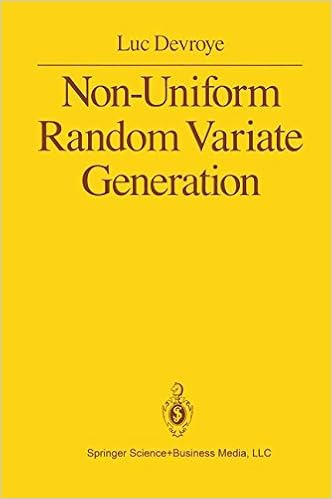## the strange occurrence of the one bump

Posted in Books, Kids, R, Statistics with tags , , , , , , , , on June 8, 2020 by xi'anWhen answering an X validated question on running an accept-reject algorithm for the Gamma distribution by using a mixture of Beta and drifted (bt 1) Exponential distributions, I came across the above glitch in the fit of my 10⁷ simulated sample to the target, apparently displaying a wrong proportion of simulations above (or below) one.

a=.9
g<-function(T){
x=rexp(T)
v=rt(T,1)<0
x=c(1+x[v],exp(-x/a)[!v])
x[runif(T)<x^a/x/exp(x)/((x>1)*exp(1-x)+a*(x<1)*x^a/x)*a]}

It took me a while to spot the issue, namely that the output of

  z=g(T)
while(sum(!!z)<T)z=c(z,g(T))
z[1:T]


was favouring simulations from the drifted exponential by truncating. Permuting the elements of z before returning solved the issue (as shown below for a=½)!## ratio-of-uniforms [#3]

Posted in Books, pictures, R, Statistics with tags , , , , , on November 4, 2016 by xi'anBeing still puzzled (!) by the ratio-of-uniform approach, mostly failing to catch its relevance for either standard distributions in a era when computing a cosine or an exponential is negligible, or non-standard distributions for which computing bounds and boundaries is out-of-reach, I kept searching for solutions that would include unbounded densities and still produce compact boxes, as this seems essential for accept-reject simulation if not for slice sampling. And after exploring some dead-ends (in tune with running in Venezia!), I came upon the case of the generalised logistic transform$h(\omega)=\omega^a/(1+\omega^a)$

which ensures that the [ratio-of-almost-uniform] set I defined in my slides last week$\mathfrak{H}=\left\{(u,v);\ 0\le u\le h(f(v/g(u))\right\}$

is bounded in u. Since the transform g is the derivative of the inverse of h (!),$g(y)=a^{-1}y^{(1-a)/a}/(1-y)^{(1-3a)/a}$

the parametrisation of the boundary of H is$u(x)=f(x)^a/(1+f(x)^a)\ v(x)=a^{-1}xf(x)^{(a-1)/a}(1+f(x)^a)^2$

which means it remains bounded if (a) a≤1 [to ensure boundedness at infinity] and (b) the limit of v(x) at zero [where I assume the asymptote stands] is bounded. Meaning$\lim_{x\to 0} xf(x)^{2a+1/a-1}<\infty$

Working a wee bit more on the problem led me to realise that resorting to an arbitrary cdf Φ instead of the logistic cdf could solve the problem for most distributions, including all Gammas! Indeed, the boundary of H is now$u(x)=\Phi(f(x))^a\ v(x)=a^{-1}xf(x)^{(a-1)/a}/\varphi(f(x))$

which means it remains bounded if φ has very heavy tails, like 1/x². To handle the explosion when x=0. And an asymptote itself at zero, to handle the limit at infinity when f(x) goes to zero.